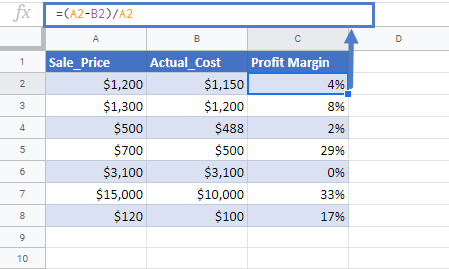# Profit Margin Calculator in Excel & Google Sheets

This tutorial will demonstrate how to calculate the percentage profit margin in Excel & Google Sheets.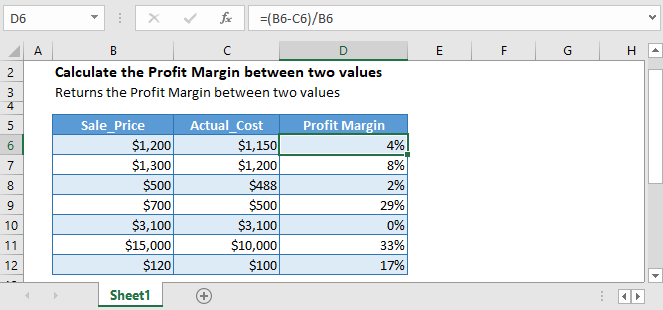## Profit Margin

The profit margin indicates how much a company makes in profit from a sale. It represents what percentage of sales has turned into profit.

## Calculate Profit Margin from the table

Let’s say that you a list of data with Sale Price and Actual Cost of the Products. Then the profit margin will be calculated by subtracting the actual cost from the sale price and then dividing it by the sale price, like this:

``=(B3-C3)/B3``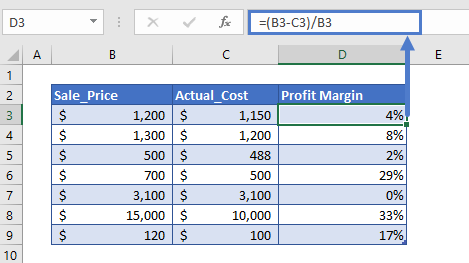The formula can easily be understood by breaking it down into the following 4 simple steps:

### Step 1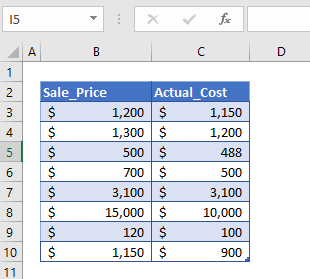First of all, we need to prepare the data for the calculation of the profit margin. Here, in the above table, we’ve put some values that we are going to use to find the profit margin between the sale_price and actual_cost.

### Step 2

After preparing the data, we’ll calculate the profit for the values. The profit is simply calculated by subtracting the column C values from column B values.

``=B3-C3``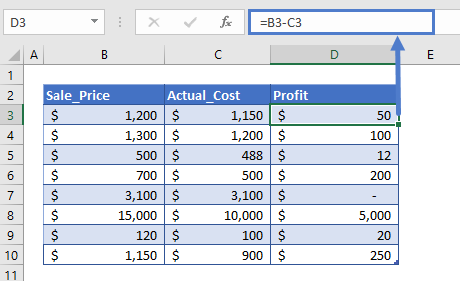The difference we calculated can have a positive value or negative value, meaning the change in value can be positive and negative.

### Step 3

Now we simply divide the profit value or change in value (column D) with the sale_price (column B). This will give us the result in number format

``=D3/B3``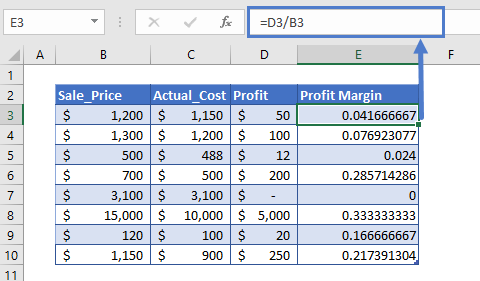### Step 4

The result we get in step 3 is in number format. We need the values in the percentage form. So, we’ll change the number format of the entire column E. To do so,

1. First, we’ll select the cells from E3 to E10.
2. Then on the Home tab, in the Number group, click the % icon for changing the number format to Percent Style.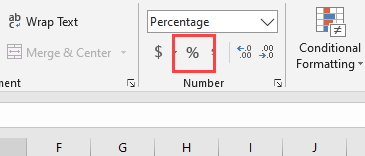After this, we’ll get the profit margin in percentage between the two numbers.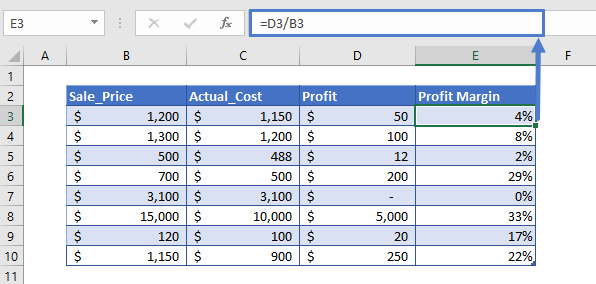### Easy Excel Automation